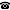# 2005 seminar talk: Continuous Fraisse conjecture and the number of Gödel logics

Talk held by Martin Goldstern (TU Wien) at the KGRC seminar on 2005-01-18.

### Abstract

Linear orders are naturally quasiordered by embeddability. Answering a question of Fraisse, Laver showed that this quasiorder, restricted to the scattered linear orders (those that do not contain a copy of the rationals), is a well-quasi-order; he also showed that there are exactly aleph1 equivalence classes (modulo bi-embeddability) of countable linear orders. In a joint paper with Arnold Beckmann and Norbert Preining we generalize this theorem to the natural quasiorder that is given by CONTINUOUS embeddability. A Gödel logic is given by a closed subset G of the unit intervall (containing 0 and 1). Fuzzy (relational) G-models are sets M with maps M^k -> G for every k-ary predicate symbol. A fuzzy satisfaction function is defined naturally; the "Gödel logic" associated with G is the set of all sentences which have value 1 in every fuzzy G-model. All these logics are contained in the set of classical validities; as an application of the continuous Fraisse conjecture, we show that there are only countably many Gödel logics.

Kurt Gödel Research Center for Mathematical Logic. Währinger Straße 25, 1090 Wien, Austria.+43-1-4277-50501. Last updated: 2010-12-16, 04:37.